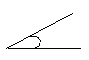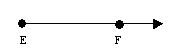Name: ___________________Date:___________________

 Email us to get an instant 20% discount on highly effective K-12 Math & English kwizNET Programs!

### Grade 4 - Mathematics4.10 Lines and Angles Review Test

 Q 1: A triangle with all angles equal is calledobtuse triangleequiangular triangleacute triangle Q 2: An angle that is less than a right angle isangle lessacute anglea right angle Q 3: Which of these figures is not a quadrilateralfigure 2figure 1figure 3 Q 4: A four sided figure with all equal sides is called apentagonsquarenonagon Q 5: The angle WXY is an acute angle.TrueFalse Q 6: An acute angle isLess than 90Greater than 90Equal to 90 Q 7: A triangle with all sides equal is calledscalene triangleisosceles trianglean equilateral triangle Q 8: A part of a line is____pointrayplane Question 9: This question is available to subscribers only! Question 10: This question is available to subscribers only!